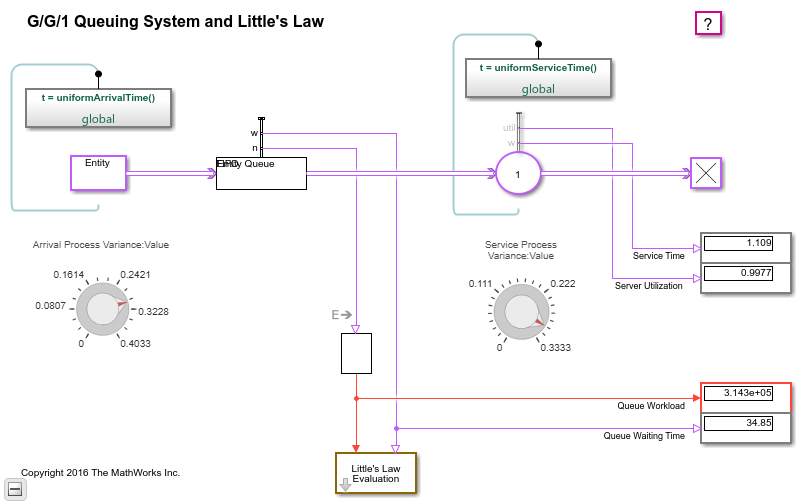# G/G/1 Queuing System and Little's Law

### Overview

This example shows how to model a single-queue single-server system in which the interarrival time and the service time are uniformly distributed with fixed means of 1.1 and 1, respectively. The queue has an infinite storage capacity. In the notation, the G stands for a general distribution with a known mean and variance; G/G/1 means that the system's interarrival and service times are governed by such a general distribution, and that the system has one server. You can change the variances of the uniform distributions. You can use this model to examine Little's law.

### Structure of the Model

The model includes the components listed below:

• Entity Generator block: Generates entities (also known as "customers" in queuing theory).

• Simulink Function uniformArrivalTime(): Returns data representing the interarrival times for the generated entities. After you set the distribution's variance using the Arrival Process Variance knob, the function computes a uniform random variate with the chosen variance and mean 1.1. To see the computation details, double-click the Simulink Function and open the block labeled Uniform Distribution.

• Entity Queue block: Stores entities that are to be served in FIFO order

• Entity Server block: Models a server whose service time has a uniform distribution.### Results and Displays

The model includes these visual ways to understand its performance:

• Display blocks that show the queue workload, average waiting time in the queue, average service time, and server utilization.

• A scope comparing empirical and theoretical ratios. See the discussion of Little's law below.### Little's Law

You can use this model to verify Little's law, which states the linear relationship between average queue length and average waiting time in the queue. In particular, the expected relationship is as follows:

Average queue length = (Mean arrival rate)(Average waiting time in queue)

The Entity Queue block computes the current queue length and average waiting time in the queue. The subsystem called Little's Law Evaluation computes the ratio of average queue length (derived from the instantaneous queue length via integration) to average waiting time, as well as the ratio of mean service time to mean arrival time. The two ratios appear on the plot labeled Little's Law.

Another way to interpret the equation above is that, given a normalized mean service time of 1, you can use the average waiting time and average queue length to derive the system's arrival rate.

### Little's Law Applied to the Server

You can also use this model to verify the linear relationship that Little's law predicts between the server utilization and the average service time. The Entity Server block computes the server utilization and average waiting time in the server. Because each entity can depart from the server immediately upon completing service, waiting time is equivalent to service time for the server in this model.

### Experimenting with the Model

Move the Arrival Process Variance knob or the Service Process Variance knob during the simulation and observe how the queue content changes. When traffic intensity is high, the average waiting time in the queue is approximately linear in the variances of the interarrival time and service time. The larger the variances are, the longer an entity has to wait, and the more entities are waiting in the system.

### References

 Kleinrock, Leonard, Queueing Systems, Volume I: Theory, New York, Wiley, 1975.